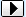MENU Request Lyrics   Add/Correct Lyrics   Links Page   Contact Us   Privacy Policy   DMCA Policy MERCHANDISE Country CDs   Country Sheet MusicBROWSE LYRICS AND TABS BY ARTIST/BAND NAME:SEARCH:A B C D E F G H I J K L M N O P Q R S T U V W X Y Z ## Do the Math lyrics - George FoxYou talk about her like she's not even gone,
And you act like any minute, she might call.
But i was there when you got that dear john letter,
So if you don't think it's over, boy you better.

Do the math,
That girl ain't coming back,
Do the math,
Divide and then subtract,
Multiply the pain you're heading for,
If you wanna know the score,
Do the math.

You've always complicated everything,
And now you're left with too much time to think.
You're still looking for a common denominator,
You don't see her now,
And you won't see her, later.

Do the math,
That girl ain't coming back,
Do the math,
Divide and then subtract,
Multiply the pain you're heading for,
If you wanna know the score,
Do the math.

You must've calculated something wrong,
'X' only knows just 'y' she's gone.
Do the math.

Do the math,
That girl ain't coming back,
Do the math,
Divide and then subtract,
Multiply the pain you're heading for,
If you wanna know the score,

Do the math,
That girl ain't coming back,
Do the math,
Divide and then subtract,
Multiply the pain you're heading for,
If you wanna know the score,
She's not coming back anymore,
Do the math.

Do the math.

Fox George Chords

Fox George Chords